## 原始持仓分析

universe = [‘FB’, ‘GOOG’, ‘AMZN’, ‘AAPL’]

weights = np.array([0.25, 0.25, 0.25, 0.25])

prices = get_pricing(universe, fields=‘price’, start_date=start, end_date=end)

normal_prices = (prices-prices.min())/(prices.max()-prices.min())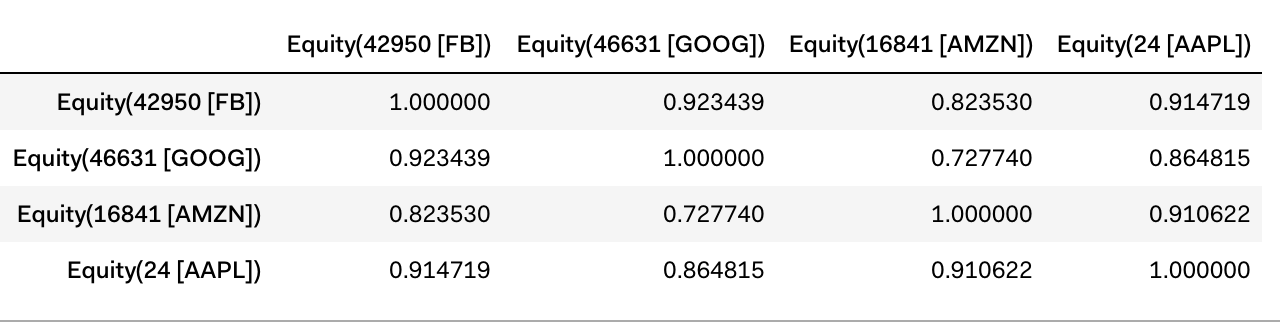sns.heatmap(cov,xticklabels=cov.columns,yticklabels=cov.columns)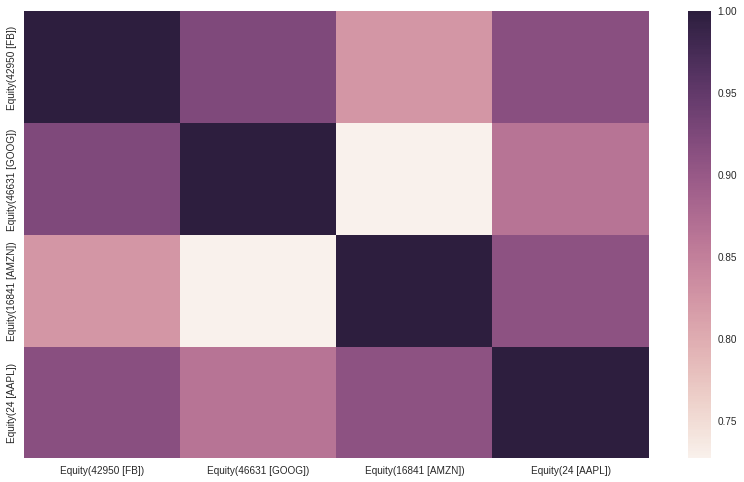total_variance = np.dot(np.dot(weights, cov_matrix.values), weights.T)

## 原始持仓+TSLA

universe_T = [‘FB’, ‘GOOG’, ‘AMZN’, ‘AAPL’, ‘TSLA’] weights_T = np.array([0.2, 0.2, 0.2, 0.2, 0.2])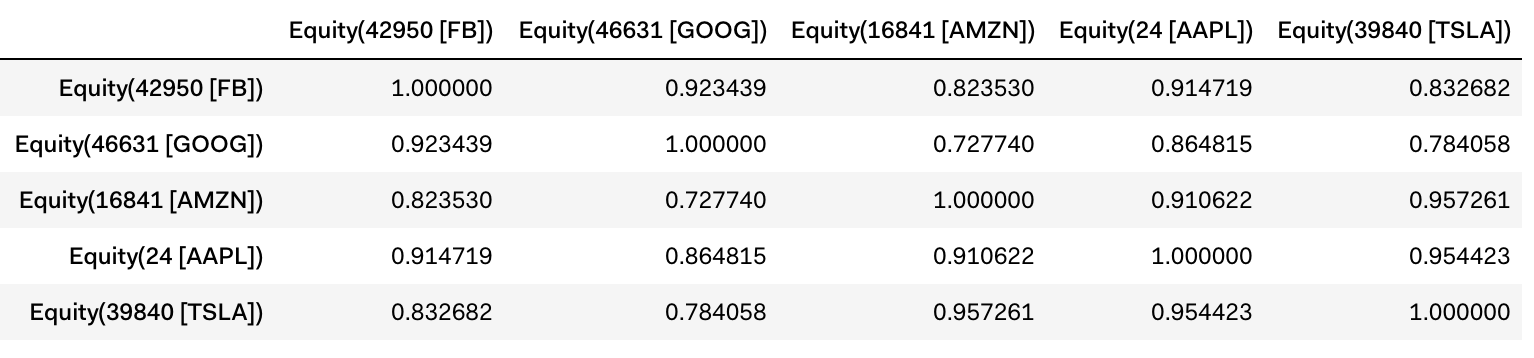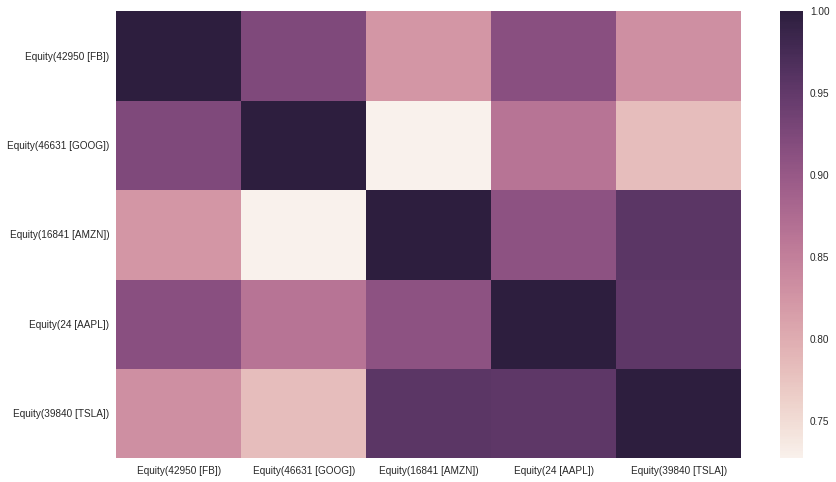## 原始持仓+BRK.B

universe_B = [‘FB’, ‘GOOG’, ‘AMZN’, ‘AAPL’, ‘BRK.B’] weights_B = np.array([0.2, 0.2, 0.2, 0.2, 0.2])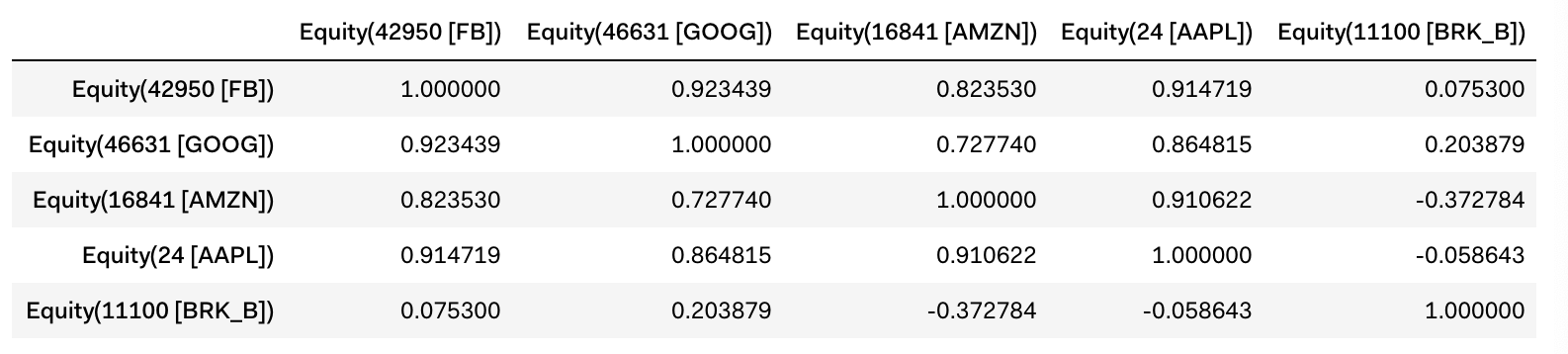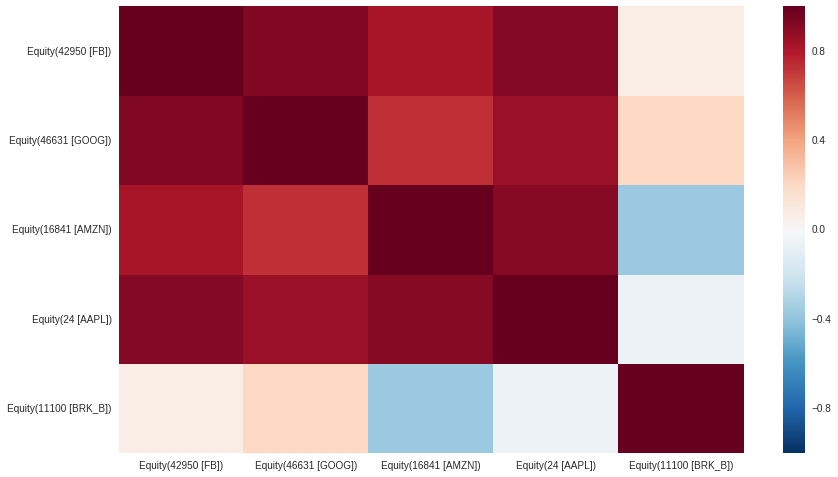https://www.quantopian.com/lectures/linear-correlation-analysis

https://www.quantopian.com/lectures/position-concentration-risk